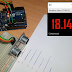This article shows how to design a distance measuring system with a Graphical User Interface App on a computer to display the measured values. The system uses Arduino uno and HC-SR04 ultrasonic sensor. The App was built using Java (JavaFx). you can download the source code at the end of this page.

This video demonstrate how to setup and design the system

First of all the measuring system consist of a ultrasonic sensor and a microcontroller for the signal processing and serial communication.

## Ultrasonic sensors

• Uses sonar to determine distance
• Can measure distance between 2 cm to 400cm
• If we know the time (t) it took the signal to hit the object and bounced back
• We can calculate the distance by
$d= \text{velocity of sound}(v)\times\frac{t}{2}$
• Velocity of sound in air is $$34300 \ cm/s$$ or $$0.0343 \ cm/\mu s$$
we will be measuring time in microseconds($$\mu s = 1\times 10^{-6} seconds$$)
$d=\frac{0.0343t}{2} \text{ cm}$ where t is in microseconds
$d=0.01715\times t \ (cm))$
this implies that when we measure the time (in microseconds) it took the signal to be transmitted and received we will just multiply it by 0.01715 to get the distance
The firmware design is simple we just measure the distance and transmit it via the serial port. we do this approximately every 0.4 seconds or 400 ms

### Software Design

we going to design a simple GUI that will accept this transmitted data from the serial port and display it nicely.
On the software side when the Arduino board is connected to the PC we list the available ports in a combo box the user will then have to select the port the Board is connected to and then click the connect button. After this step is successful  the software will read the data being sent by the board and then displays it.

We designed this software using JavaFx and JSerial library

Distance Measurement System with JavaFx GUI using Arduino and Ultrasonic SensorReviewed by Zakaria Mohammed on July 22, 2019 Rating: 5

#### 1 comment :

1.I would be happy to learn how the system works. I would highly value your answer! Thank you in advance for helping us!